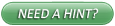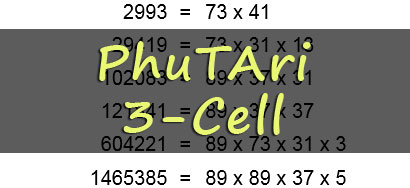## PhuTAri Puzzles

Rulewise, PhuTAri is deceptively simple. Your job is to distribute all of the numbers in the top section of the puzzle among the 2 or 3 rectangular cells according to one rule: The product of all of the numbers in each cell must be the same.

In other words, you must distribute all of the numbers among the cells so that multiplying all of the numbers in one cell gives the same result as multiplying all of the numbers in any other cell. For an unrealistically simple example, if the numbers were 2, 4, 8, and 4, we would put 2 and 8 in one cell, and we would put 4 and 4 in the other cell. (2x8=16, 4x4=16)

Below the cells is a list of the Prime Factorizations of all of the numbers in the puzzle. You can use this information to determine where to place each number, because the "Fundamental Theorem of Arithmetic" dictates that in order for the final products to be equal, the exact same amount of each prime number will end up in each master cell.

Do not be fooled, this game is won by using Logic. You don't have to do multiplication, division, or arithmetic of any kind to win.

A new PhuTAri puzzle will be offered here each day. Puzzles are created and copyrightd by Tim Clark.## Phutari 2-Cell

Move each of the numbers provided into one of two master cells, in such a way so that the product of all the numbers in one cell equals the product of all the numbers in the other.## Phutari 3-Cell

Move each of the numbers provided into one of three master cells, in such a way so that the product of all the numbers in each master cell equals the product of all the numbers in each other master cell.